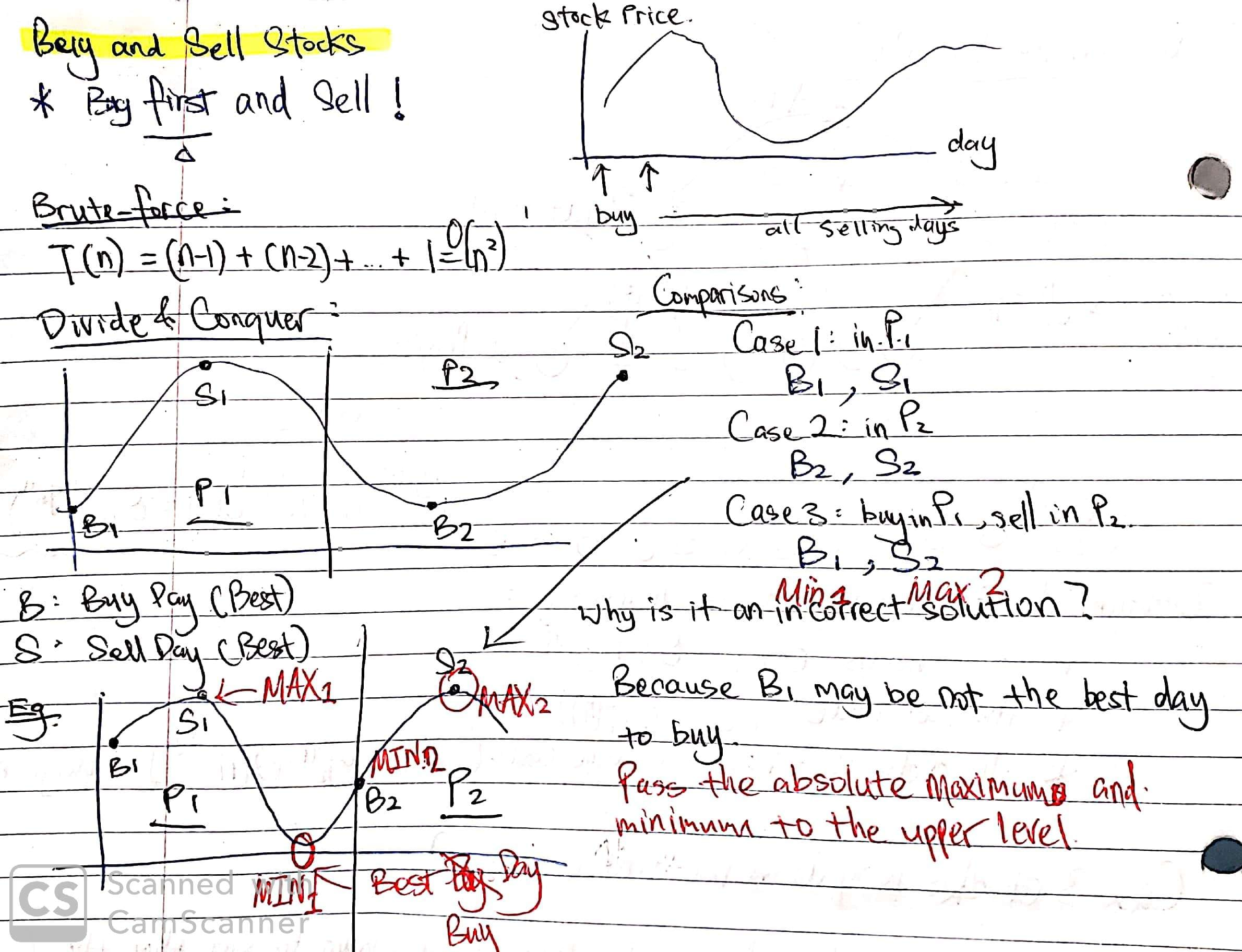# 121. Best Time to Buy and Sell Stock

Reference: LeetCode
Difficulty: Easy

## Problem

Say you have an array for which the ith element is the price of a given stock on day i.

If you were only permitted to complete at most one transaction (i.e., buy one and sell one share of the stock), design an algorithm to find the maximum profit.

Constraint: Note that you cannot sell a stock before you buy one.

Example:

## Analysis

### Brute-Force

For each day i, compare its prices with the following price of each day j, and update the maximum profit.

Note:

• j should starts from 0.
• maxProfit should be initialized with $0$.

Time: $O(N^2)$
Space: $O(1)$

### Divide and Conquer

We divide the problem into two subproblems left and right. Then we calculate:

• The best buy time B1 and the best sell time S1 from left.
• The best buy time B2 and the best sell time S2 from right.
• The time M1 of minimum price and the time X1 of maximum price in left.
• The time M2 of minimum price and the time X2 of maximum price in right.

In the combine step, we have three cases to consider:

• Case 1: In left, we buy at B1 and sell at S1.
• Case 2: In right, we buy at B2 and sell at S2.
• Case 3: In left and right, we buy at M1 and sell at X2.
• We cannot use B1 and S2 because B1 may not be the time of minimum price and S2 may not be the time of maximum price. Check out the illustration below.
• Beside, we need to update the time of minimum price and maximum price.

Here is an illustration of the text above. Notice the left is P1 and right is P2.The following solution uses a helper class BuySell to encapsulate variables passed across recursive levels.

Here is the main part of the solution. Notice that it is a good approach to separate the combining code from the recursive function. It makes code more readable.

Time: $O(N)$ since $T(N) = 2T(N) + O(1)$
Space: $O(\log{N})$ because of the call stack.

### One-Pass

Notice that the maximum profit that can be made by selling on a specific day is determined by the minimum of the stock prices over the previous days. Thus, we can loop over each price in prices, and keep updating the minimum price and at the same update the maximum profit.

Note: The ordering of updating maxProfit and minPrice does not matter.

Time: $O(N)$
Space: $O(1)$

CommentJunhao Wang
a software engineering cat